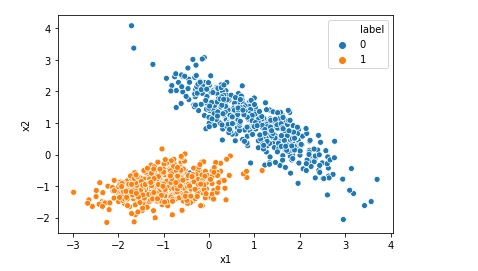# Logistic Regression Machine Learning Algorithm from Scratch in Python

Logistic Regression Machine Learning Algorithm From Scratch in Python is a short video course to discuss an overview of the Logistic Regression Machine Learning Algorithm. In this video different types of concepts related to Logistic Regression Algorithms such as Sigmoid and Optimization are discussed. Finally, the algorithm is implemented, step by step, in Python Programming … Continue reading Logistic Regression Machine Learning Algorithm from Scratch in Python# Creating Synthetic Data for Logistic Regression

Many a times we want to implement Logistic Regression on certain data but we do not find that kind of data online. In that case we can generate a synthetic data for our problem. In this post we will see how to generate a typical synthetic data for a simple Logistic Regression. Import the required … Continue reading Creating Synthetic Data for Logistic Regression# What is Confusion Matrix in Machine Learning

Not only human beings but also the machine learning models may get confused !! After all Artificial Intelligence mimics a human brain, isn’t it?(pun intended). Imagine yourself as a machine learning engineer and suppose you trained a machine learning classification model successfully today. After the model is trained you checked the accuracy which is 93.0%. Wow … Continue reading What is Confusion Matrix in Machine Learning# Difference between Logistic Regression and Support Vector Machine SVM

Both Logistic Regression and Support Vector Machine or SVM can be used for classification however there is some fundamental difference between them. Lets understand those differences when we are using Support Vector Machine as a classifier. Logistic Regression gives probabilities as output that can be interpreted as confidence in the classification decision however Support Vector … Continue reading Difference between Logistic Regression and Support Vector Machine SVM

# Top 5 Hyper-Parameters for Logistic Regression

Hyper-parameter is a type of parameter for a machine learning model whose value is set before the model training process starts. Most of the algorithm including Logistic Regression deals with useful hyper parameters. In this post we are going to discuss about the sklearn implementation of hyper-parameters for Logistic Regression. Below is the list of … Continue reading Top 5 Hyper-Parameters for Logistic Regression# Implementing Logistic Regression in 10 lines in Python

Logistic Regression is one of the most popular Machine Learning algorithm used for the classification problems. It should be noted that though there is a regression word in the name of the algorithm Logistic Regression, it is used for classification problems. A use case of Logistic regression could be, based on the symptoms for a disease that a patient has Logistic … Continue reading Implementing Logistic Regression in 10 lines in Python